# How to wrangle log data with Python and Apache Spark

## Case study with NASA logs to show how Spark can be leveraged for analyzing data at scale.Image by :
Opensource.com

## Subscribe now

Get the highlights in your inbox every week.

One of the most popular and effective enterprise use-cases which leverage analytics today is log analytics. Nearly every organization today has multiple systems and infrastructure running day in and day out. To effectively keep their business running, these organizations need to know if their infrastructure is performing to its maximum potential. Finding out involves analyzing system and application logs and maybe even applying predictive analytics on log data. The amount of log data involved is typically massive, depending on the type of organizational infrastructure involved and applications running on it.

## processing_and_wrangling_nasa_logs_figure_1_500.jpg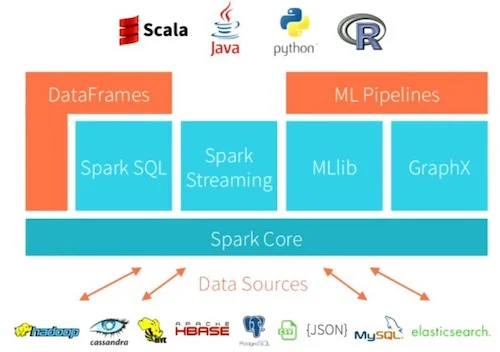The log data processing pipeline.

Gone are the days when we were limited to analyzing a data sample on a single machine due to compute constraints. Powered by big data, better and distributed computing, and frameworks like Apache Spark for big data processing and open source analytics, we can perform scalable log analytics on potentially billions of log messages daily. The intent of this case study-oriented tutorial is to take a hands-on approach showcasing how we can leverage Spark to perform log analytics at scale on semi-structured log data. If you are interested in scalable SQL with Spark, feel free to check out SQL at scale with Spark.

While there are many excellent open source frameworks and tools out there for log analytics—such as Elasticsearch—the intent of this two-part tutorial is to showcase how Spark can be leveraged for analyzing logs at scale. In the real world, you are of course free to choose your own toolbox when analyzing your log data.

Let’s get started!

## Main objective: NASA log analytics

As we mentioned before, Apache Spark is an excellent and ideal open source framework for wrangling, analyzing and modeling structured and unstructured data—at scale! In this tutorial, our main objective is one of the most popular use-cases in the industry—log analytics. Server logs are a common enterprise data source and often contain a gold mine of actionable insights and information. Log data comes from many sources in these conditions, such as the web, client and compute servers, applications, user-generated content, and flat files. These logs can be used for monitoring servers, improving business and customer intelligence, building recommendation systems, fraud detection, and much more.

Spark allows you to cheaply dump and store your logs into files on disk, while still providing rich APIs to perform data analysis at scale. This hands-on case study will show you how to use Apache Spark on real-world production logs from NASA while learning data wrangling and basic yet powerful techniques for exploratory data analysis. In this study, we will analyze log datasets from the NASA Kennedy Space Center web server in Florida.

The full data set—containing two months’ worth of all HTTP requests to the NASA Kennedy Space Center—is freely available here for download. Or, if you prefer FTP:

Next, if you want to follow along, download the tutorial from my GitHub and place both of these files in the same directory as the tutorial’s Jupyter Notebook.

## Setting up dependencies

The first step is to make sure you have access to a Spark session and cluster. For this step, you can use your own local Spark setup or a cloud-based setup. Typically, most cloud platforms provide a Spark cluster these days and you also have free options, including Databricks community edition. This tutorial assumes you already have Spark set up, hence we will not be spending additional time configuring or setting up Spark from scratch.

Often pre-configured Spark setups already have the necessary environment variables or dependencies pre-loaded when you start your Jupyter Notebook server. In my case, I can check them using the following commands in my notebook:

``spark``

## spark_session.png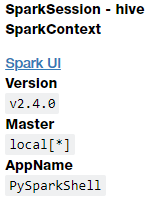These results show me that my cluster is running Spark 2.4.0 at the moment. We can also check if sqlContext is present using the following code:

``sqlContext``

`<pyspark.sql.context.SQLContext at 0x7fb1577b6400>`

Now in case you don’t have these variables pre-configured and get an error, you can load and configure them using the following code:

```# configure spark variables
from pyspark.context import SparkContext
from pyspark.sql.context import SQLContext
from pyspark.sql.session import SparkSession

sc = SparkContext()
sqlContext = SQLContext(sc)
spark = SparkSession(sc)

import re
import pandas as pd```

We also need to load other libraries for working with DataFrames and regular expressions. Working with regular expressions is one of the major aspects of parsing log files. This tool offers a powerful pattern-matching technique which can be used to extract and find patterns in semi-structured and unstructured data.

## perl_problems_600.pngThe Perl Problems strip from xkcd.

Regular expressions can be extremely effective and powerful, yet they can also be overwhelming and confusing. Not to worry though, with practice you can really leverage their maximum potential. The following example showcases a way of using regular expressions in Python. Here, we try to find all occurences of the word 'spark' in a given input sentence.

```m = re.finditer(r'.*?(spark).*?', "I'm searching for a spark in PySpark", re.I)
for match in m:
print(match, match.start(), match.end())```

```<_sre.SRE_Match object; span=(0, 25), match=“I’m searching for a spark”> 0 25 <_sre.SRE_Match object; span=(25, 36), match=’ in PySpark’> 25 36```

Let’s move on to the next part of our analysis.

Given that our data is stored in the following path (in the form of flat files), let’s load it into a DataFrame. We’ll do this in steps. The following code loads our disk’s log data file names:

```import glob

raw_data_files = glob.glob('*.gz')
raw_data_files```

`[‘NASA_access_log_Jul95.gz’, ‘NASA_access_log_Aug95.gz’]`

Now, we’ll use sqlContext.read.text() or spark.read.text() to read the text file. This code produces a DataFrame with a single string column called value:

```base_df = spark.read.text(raw_data_files)
base_df.printSchema()```

```root |-- value: string (nullable = true)```

This output allows us to see the text for our log data’s schema that we will soon inspect. You can view the type of data structure holding our log data using the following code:

``type(base_df)``

`pyspark.sql.dataframe.DataFrame`

Throughout this tutorial we use Spark DataFrames. However if you want, you can also convert a DataFrame into a Resilient Distributed Dataset (RDD)—Spark’s original data structure ()—if needed by adding the following code:

```base_df_rdd = base_df.rdd
type(base_df_rdd)```

`pyspark.rdd.RDD`

Let’s now take a peek at the actual log data in our DataFrame:

``base_df.show(10, truncate=False)``

## processing_and_wrangling_nasa_logs_figure_4_600.png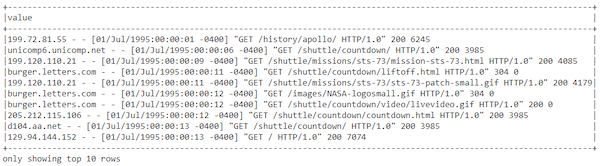The log data within the dataframe.

This result definitely looks like standard semi-structured server log data. We will definitely need to do some data processing and wrangling before this file is useful. Do remember that accessing data from RDDs is slightly different as seen below:

``base_df_rdd.take(10)``

## processing_and_wrangling_nasa_logs_figure_5_600.pngLog data from the resilient distributed datasets.

Now that we have loaded and viewed our log data, let’s process and wrangle it.

## Data Wrangling

In this section, we clean and parse our log dataset to extract structured attributes with meaningful information from each log message.

### Log data understanding

If you’re familiar with web server logs, you’ll recognize that the data displayed above is in Common Log Format. The fields are:`  `

`remotehost rfc931 authuser [date] "request" status bytes`

Field Description
remotehost Remote hostname (or IP number if DNS hostname is not available or if DNSLookup is off).
rfc931 The remote logname of the user if at all it is present.
authuser The username of the remote user after authentication by the HTTP server.
[date] Date and time of the request.
“request” The request, exactly as it came from the browser or client.
status The HTTP status code the server sent back to the client.
bytes The number of bytes (`Content-Length`) transferred to the client.

We now need techniques to parse, match, and extract these attributes from the log data.

### Data parsing and extraction with regular expressions

Next, we have to parse our semi-structured log data into individual columns. We’ll use the special built-in regexp_extract() function to do the parsing. This function matches a column against a regular expression with one or more capture groups, and allows you to extract one of the matched groups. We’ll use one regular expression for each field we wish to extract.

You must have heard or used a fair bit of regular expressions by now. If you find regular expressions confusing (and they certainly can be), and you want to learn more about them, we recommend checking out the RegexOne web site. You might also find Regular Expressions Cookbook, by Goyvaerts and Levithan, to be a useful reference.

Let’s take a look at the total number of logs we are working within our dataset:

``print((base_df.count(), len(base_df.columns)))``

`(3461613, 1)`

Looks like we have a total of approximately 3.46 million log messages. Not a small number! Let’s extract and take a look at some sample log messages:

```sample_logs = [item['value'] for item in base_df.take(15)]
sample_logs```

### Extracting hostnames

Let’s write some regular expressions to extract the hostname from the logs:

```host_pattern = r'(^\S+\.[\S+\.]+\S+)\s'
hosts = [re.search(host_pattern, item).group(1)
if re.search(host_pattern, item)
else 'no match'
for item in sample_logs]
hosts```

```[‘199.72.81.55’, ‘unicomp6.unicomp.net’, ‘199.120.110.21’, ‘burger.letters.com’, …, …, ‘unicomp6.unicomp.net’, ‘d104.aa.net’, ‘d104.aa.net’]```

### Extracting timestamps

Let’s use regular expressions to extract the timestamp fields from the logs:

```ts_pattern = r'\[(\d{2}/\w{3}/\d{4}:\d{2}:\d{2}:\d{2} -\d{4})]'
timestamps = [re.search(ts_pattern, item).group(1) for item in sample_logs]
timestamps```

```[‘01/Jul/1995:00:00:01 -0400’, ‘01/Jul/1995:00:00:06 -0400’, ‘01/Jul/1995:00:00:09 -0400’, …, …, ‘01/Jul/1995:00:00:14 -0400’, ‘01/Jul/1995:00:00:15 -0400’, ‘01/Jul/1995:00:00:15 -0400’]```

### Extracting HTTP request method, URIs, and protocol

Let’s now use regular expressions to extract the HTTP request methods, URIs, and Protocol patterns fields from the logs:

```method_uri_protocol_pattern = r'\"(\S+)\s(\S+)\s*(\S*)\"'
method_uri_protocol = [re.search(method_uri_protocol_pattern, item).groups()
if re.search(method_uri_protocol_pattern, item)
else 'no match'
for item in sample_logs]
method_uri_protocol```

```[(‘GET’, ‘/history/apollo/’, ‘HTTP/1.0’), (‘GET’, ‘/shuttle/countdown/’, ‘HTTP/1.0’), …, …, (‘GET’, ‘/shuttle/countdown/count.gif’, ‘HTTP/1.0’), (‘GET’, ‘/images/NASA-logosmall.gif’, ‘HTTP/1.0’)]```

### Extracting HTTP status codes

Let’s now use regular expressions to extract the HTTP status codes from the logs:

```status_pattern = r'\s(\d{3})\s'
status = [re.search(status_pattern, item).group(1) for item in sample_logs]
print(status)```

`[‘200’, ‘200’, ‘200’, ‘304’, …, ‘200’, ‘200’]`

### Extracting HTTP response content size

Let’s now use regular expressions to extract the HTTP response content size from the logs:

```content_size_pattern = r'\s(\d+)\$'
content_size = [re.search(content_size_pattern, item).group(1) for item in sample_logs]
print(content_size)```

`[‘6245’, ‘3985’, ‘4085’, ‘0’, …, ‘1204’, ‘40310’, ‘786’]`

### Putting it all together

Let’s now leverage all the regular expression patterns we previously built and use the `regexp_extract(...)` method to build our DataFrame with all of the log attributes neatly extracted in their own separate columns.

```from pyspark.sql.functions import regexp_extract

logs_df = base_df.select(regexp_extract('value', host_pattern, 1).alias('host'),
regexp_extract('value', ts_pattern, 1).alias('timestamp'),
regexp_extract('value', method_uri_protocol_pattern, 1).alias('method'),
regexp_extract('value', method_uri_protocol_pattern, 2).alias('endpoint'),
regexp_extract('value', method_uri_protocol_pattern, 3).alias('protocol'),
regexp_extract('value', status_pattern, 1).cast('integer').alias('status'),
regexp_extract('value', content_size_pattern, 1).cast('integer').alias('content_size'))
logs_df.show(10, truncate=True)
print((logs_df.count(), len(logs_df.columns)))```

## processing_and_wrangling_nasa_logs_figure_7_600.png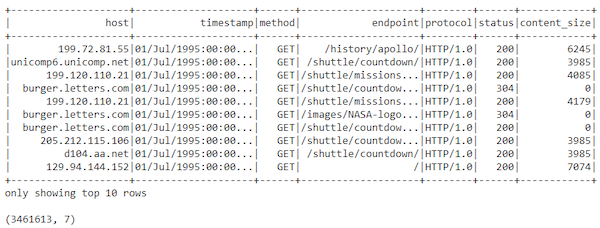Log dataframe extracted using regexp_extract(...)

### Finding missing values

Missing and null values are the bane of data analysis and machine learning. Let’s see how well our data parsing and extraction logic worked. First, let’s verify that there are no null rows in the original DataFrame:

```(base_df
.filter(base_df['value']
.isNull())
.count())```

`0`

All good! Now, if our data parsing and extraction worked properly, we should not have any rows with potential null values. Let’s try and put that to test:

```bad_rows_df = logs_df.filter(logs_df['host'].isNull()|
logs_df['timestamp'].isNull() |
logs_df['method'].isNull() |
logs_df['endpoint'].isNull() |
logs_df['status'].isNull() |
logs_df['content_size'].isNull()|
logs_df['protocol'].isNull())

`33905`

Ouch! Looks like we have over 33K missing values in our data! Can we handle this?

Do remember, this is not a regular pandas (link) DataFrame which you can directly query and get which columns have null. Our so-called big dataset is residing on disk which can potentially be present in multiple nodes in a spark cluster. So how do we find out which columns have potential nulls?

### Finding null counts

We can typically use the following technique to find out which columns have null values.

```from pyspark.sql.functions import col
from pyspark.sql.functions import sum as spark_sum

def count_null(col_name):
return spark_sum(col(col_name).isNull().cast('integer')).alias(col_name)

# Build up a list of column expressions, one per column.
exprs = [count_null(col_name) for col_name in logs_df.columns]

# Run the aggregation. The *exprs converts the list of expressions into
# variable function arguments.
logs_df.agg(*exprs).show()```

## processing_and_wrangling_nasa_logs_figure_8.png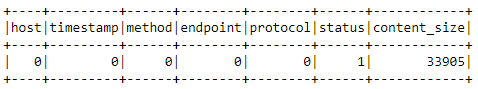Checking which columns have null values.

Well, looks like we have one missing value in the status column and everything else is in the content_size column. Let’s see if we can figure out what’s wrong!

### Handling nulls in HTTP status

Our original parsing regular expression for the status column was:

```regexp_extract('value', r'\s(\d{3})\s', 1).cast('integer')
.alias( 'status')```

Could it be that there are more digits making our regular expression wrong? Or is the data point itself bad? Let’s find out.

Note: In the expression below, the tilde (`~)` means “not”.

```null_status_df = base_df.filter(~base_df['value'].rlike(r'\s(\d{3})\s'))
null_status_df.count()```

`1`

Let’s look at what this bad record looks like:

``null_status_df.show(truncate=False)``

## processing_and_wrangling_nasa_logs_figure_9.png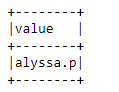A bad record that's missing information.

Looks like a record with a lot of missing information. Let’s pass this through our log data parsing pipeline:

```bad_status_df = null_status_df.select(regexp_extract('value', host_pattern, 1).alias('host'),
regexp_extract('value', ts_pattern, 1).alias('timestamp'),
regexp_extract('value', method_uri_protocol_pattern, 1).alias('method'),
regexp_extract('value', method_uri_protocol_pattern, 2).alias('endpoint'),
regexp_extract('value', method_uri_protocol_pattern, 3).alias('protocol'),
regexp_extract('value', status_pattern, 1).cast('integer').alias('status'),
regexp_extract('value', content_size_pattern, 1).cast('integer').alias('content_size'))

## processing_and_wrangling_nasa_logs_figure_10.png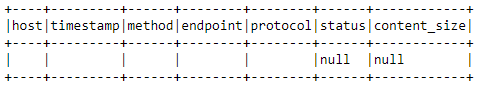The full bad log record containing no information and two null entries.

Looks like the record itself is an incomplete record with no useful information, the best option would be to drop this record as follows:

```logs_df = logs_df[logs_df['status'].isNotNull()]
exprs = [count_null(col_name) for col_name in logs_df.columns]
logs_df.agg(*exprs).show()```

## processing_and_wrangling_nasa_logs_figure_11.png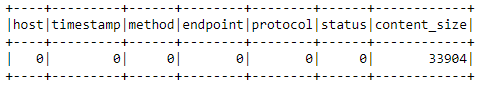The dropped record.

### Handling nulls in HTTP content size

Based on our previous regular expression, our original parsing regular expression for the content_size column was:

```regexp_extract('value', r'\s(\d+)\$', 1).cast('integer')
.alias('content_size')```

Could there be missing data in our original dataset itself? Let’s find out. We first find the records with potential missing content sizes in our base DataFrame:

```null_content_size_df = base_df.filter(~base_df['value'].rlike(r'\s\d+\$'))
null_content_size_df.count()```

`33905`

The number seems to match the number of missing content size values in our processed DataFrame. Let’s take a look at the top ten records of our data frame having missing content sizes:

``null_content_size_df.take(10)``

## processing_and_wrangling_nasa_logs_figure_12_600.png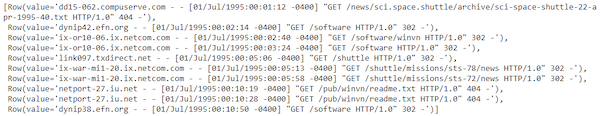The top 10 dataframe records with missing content sizes.

It is quite evident that the bad raw data records correspond to error responses, where no content was sent back and the server emitted a `-` for the `content_size` field. Since we don’t want to discard those rows from our analysis, let’s impute or fill them with 0.

### Fix the rows with null content_size

The easiest solution is to replace the null values in `logs_df` with 0 like we discussed earlier. The Spark DataFrame API provides a set of functions and fields specifically designed for working with null values, among them:

• `fillna()`, which fills null values with specified non-null values.
• `na`, which returns a `DataFrameNaFunctions` object with many functions for operating on null columns.

There are several ways to invoke this function. The easiest is just to replace all null columns with known values. But, for safety, it’s better to pass a Python dictionary containing `(column_name, value)` mappings. That’s what we’ll do. An example from the documentation is depicted below:

```>>> df4.na.fill({'age': 50, 'name': 'unknown'}).show()
+---+------+-------+
|age|height|   name|
+---+------+-------+
| 10|    80|  Alice|
|  5|  null|    Bob|
| 50|  null|    Tom|
| 50|  null|unknown|
+---+------+-------+```

Now we use this function to fill all the missing values in the `content_size` field with 0:

```logs_df = logs_df.na.fill({'content_size': 0})
exprs = [count_null(col_name) for col_name in logs_df.columns]
logs_df.agg(*exprs).show()```

## processing_and_wrangling_nasa_logs_figure_13.png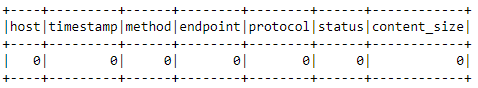The null values now replaced by zero.

Look at that, no missing values!

### Handling temporal fields (timestamp)

Now that we have a clean, parsed DataFrame, we have to parse the timestamp field into an actual timestamp. The Common Log Format time is somewhat non-standard. A User-Defined Function (UDF) is the most straightforward way to parse it:

```from pyspark.sql.functions import udf

month_map = {
'Jan': 1, 'Feb': 2, 'Mar':3, 'Apr':4, 'May':5, 'Jun':6, 'Jul':7,
'Aug':8,  'Sep': 9, 'Oct':10, 'Nov': 11, 'Dec': 12
}

def parse_clf_time(text):
""" Convert Common Log time format into a Python datetime object
Args:
text (str): date and time in Apache time format [dd/mmm/yyyy:hh:mm:ss (+/-)zzzz]
Returns:
a string suitable for passing to CAST('timestamp')
"""
# NOTE: We're ignoring the time zones here, might need to be handled depending on the problem you are solving
return "{0:04d}-{1:02d}-{2:02d} {3:02d}:{4:02d}:{5:02d}".format(
int(text[7:11]),
month_map[text[3:6]],
int(text[0:2]),
int(text[12:14]),
int(text[15:17]),
int(text[18:20])
)```

Let’s now use this function to parse our DataFrame's `time `column:

```udf_parse_time = udf(parse_clf_time)

logs_df = (logs_df.select('*', udf_parse_time(logs_df['timestamp'])
.cast('timestamp')
.alias('time'))
.drop('timestamp')
logs_df.show(10, truncate=True)```

## processing_and_wrangling_nasa_logs_figure_14_600.png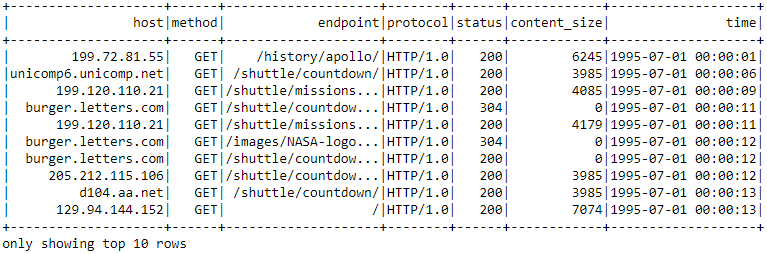The timestamp parsed with a User-Defined Function (UDF).

Things seem to be looking good! Let’s verify this by checking our DataFrame's schema:

``logs_df.printSchema()``

```root |-- host: string (nullable = true) |-- method: string (nullable = true) |-- endpoint: string (nullable = true) |-- protocol: string (nullable = true) |-- status: integer (nullable = true) |-- content_size: integer (nullable = false)|-- time: timestamp (nullable = true)```

Let’s now cache  `logs_df` since we will be using it extensively for our data analysis section in part two of this series.

``logs_df.cache()``

## Conclusion

Acquiring, processing and wrangling data are some of the most important steps in any end-to-end Data Science or Analytics use-case. Things start getting more difficult when dealing with semi-structured or unstructured data at scale. This case study gives you a step-by-step hands-on approach to leveraging the power of open-source tools and frameworks like Python and Spark to process and wrangling semi-structured NASA log data at scale. Once we have prepared a clean dataset, we can finally start using it to gain useful insights about NASA servers. Click through to the second article in this series for a hands-on tutorial on Analyzing and Visualizing NASA log data with Python and Apache Spark.

This article originally appeared on Medium's Towards Data Science channel and is republished with permission.